05 有序集合为何能同时支持点查询和范围查询？

• ZRANGEBYSCORE：按照元素权重返回一个范围内的元素。
• ZSCORE：返回某个元素的权重值。

## Sorted Set 基本结构

Sorted Set 的实现代码在t_zset.c文件中，包括 Sorted Set 的各种操作实现，同时 Sorted Set 相关的结构定义在server.h文件中。如果你想要了解学习 Sorted Set 的模块和操作，注意要从 t_zset.c 和 server.h 这两个文件中查找。

``````typedef struct zset {
dict *dict;
zskiplist *zsl;
} zset;
``````

• 跳表或是哈希表中，各自保存了什么样的数据？
• 跳表和哈希表保存的数据是如何保持一致的？

## 跳表的设计与实现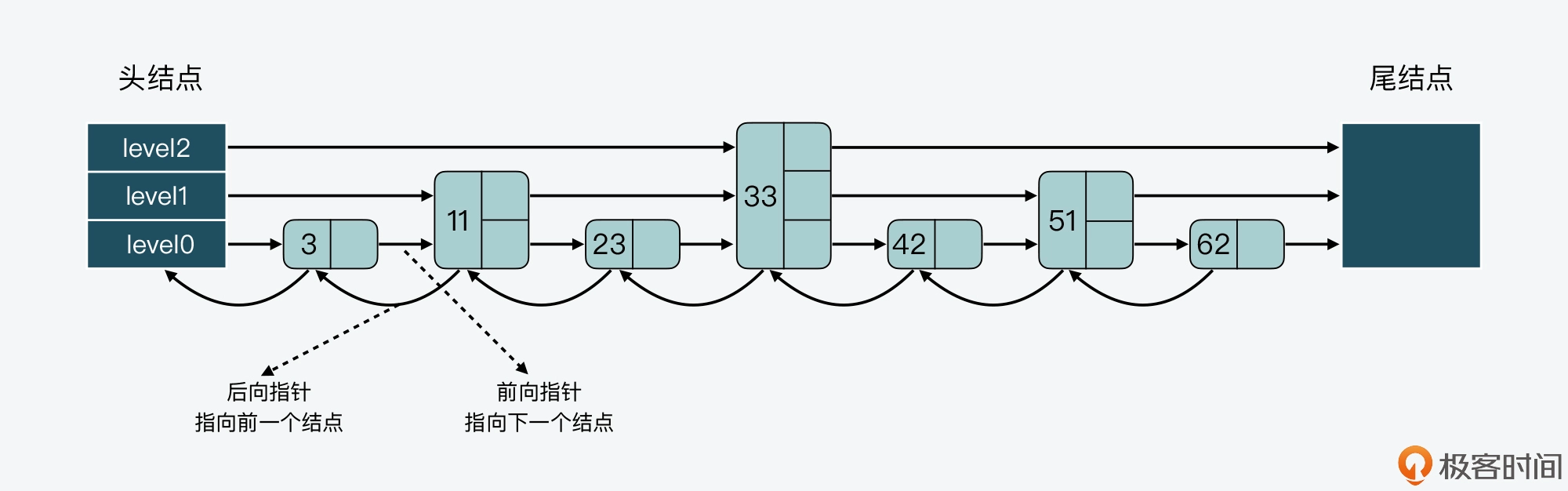### 跳表数据结构

``````typedef struct zskiplistNode {
//Sorted Set中的元素
sds ele;
//元素权重值
double score;
//后向指针
struct zskiplistNode *backward;
//节点的level数组，保存每层上的前向指针和跨度
struct zskiplistLevel {
struct zskiplistNode *forward;
unsigned long span;
} level[];
} zskiplistNode;
``````

level 数组中的每一个元素对应了一个 zskiplistLevel 结构体，也对应了跳表的一层。而 zskiplistLevel 结构体定义了一个指向下一结点的前向指针（*forward），这就使得结点可以在某一层上和后续结点连接起来。同时，zskiplistLevel 结构体中还定义了，这是用来记录结点在某一层上的*跨度forward指针和该指针指向的结点之间，跨越了 level0 上的几个结点。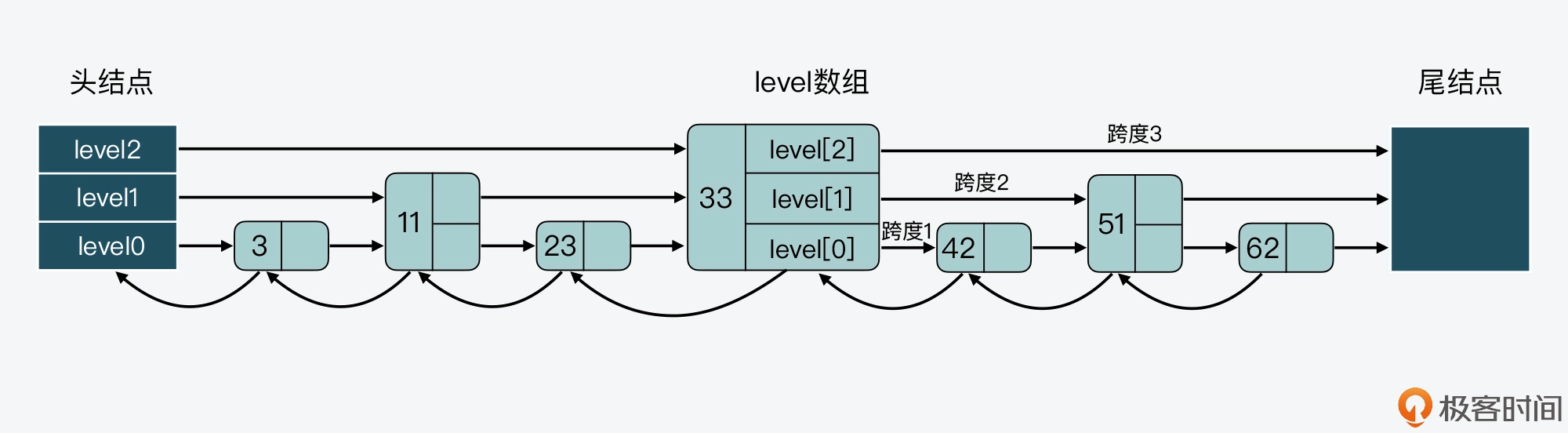``````typedef struct zskiplist {
unsigned long length;
int level;
} zskiplist;
``````

### 跳表结点查询

1. 当查找到的结点保存的元素权重，比要查找的权重小时，跳表就会继续访问该层上的下一个结点。
2. 当查找到的结点保存的元素权重，等于要查找的权重时，跳表会再检查该结点保存的 SDS 类型数据，是否比要查找的 SDS 数据小。如果结点数据小于要查找的数据时，跳表仍然会继续访问该层上的下一个结点。

``````//获取跳表的表头
//从最大层数开始逐一遍历
for (i = zsl->level-1; i >= 0; i--) {
...
while (x->level[i].forward && (x->level[i].forward->score < score || (x->level[i].forward->score == score
&& sdscmp(x->level[i].forward->ele,ele) < 0))) {
...
x = x->level[i].forward;
}
...
}
``````

### 跳表结点层数设置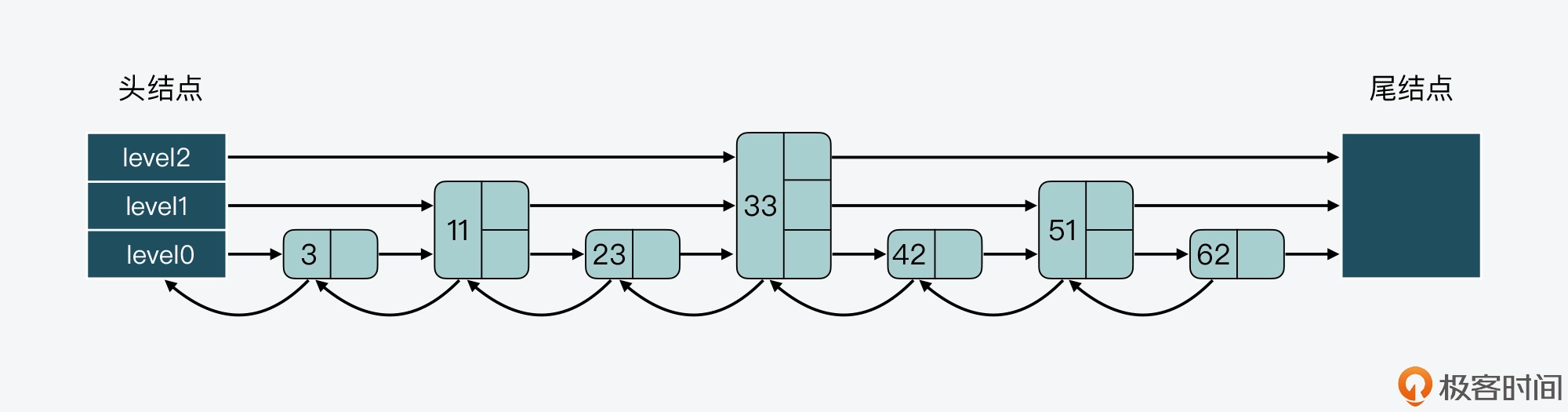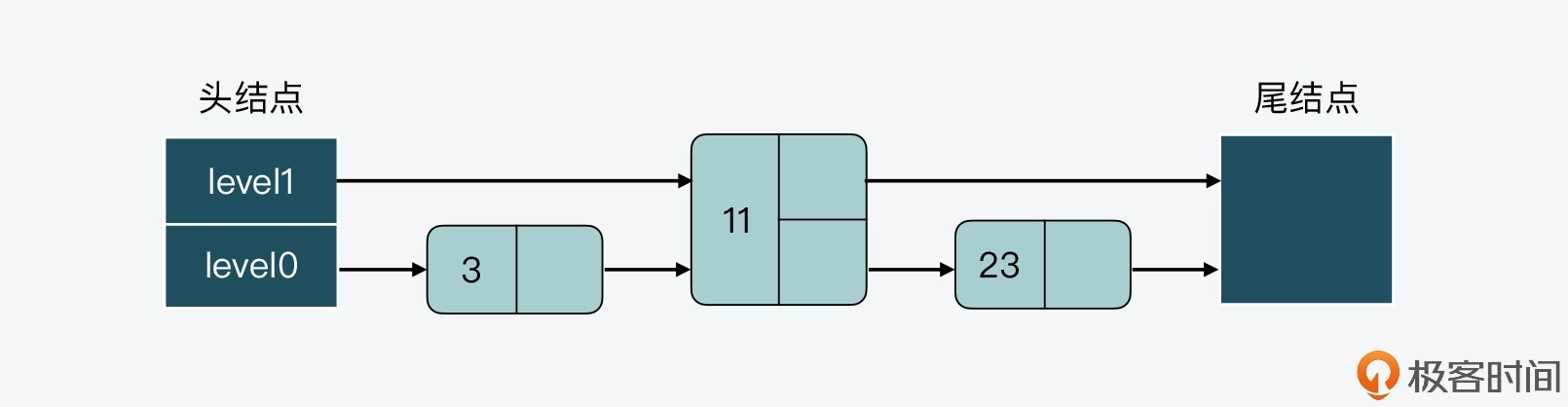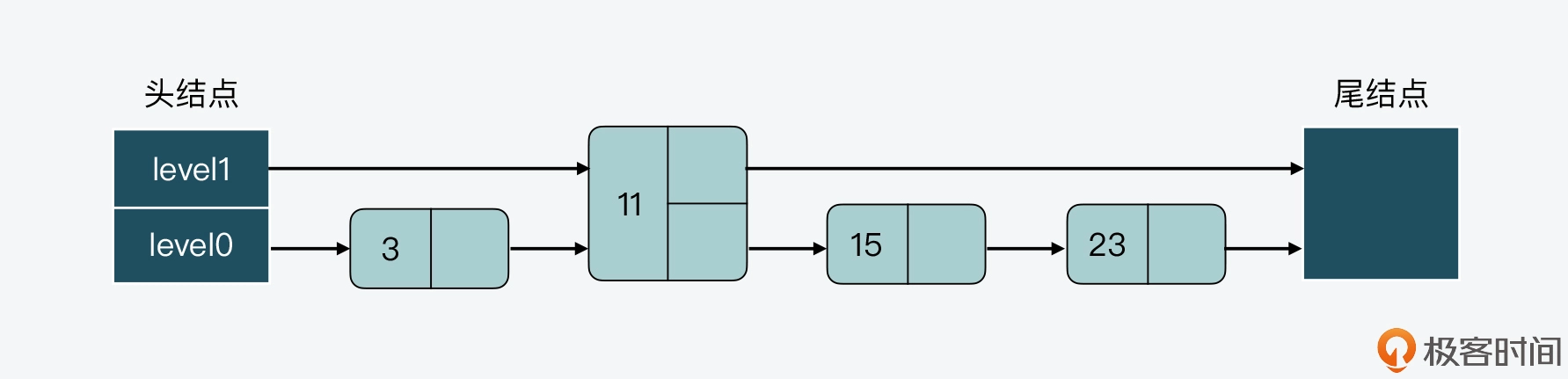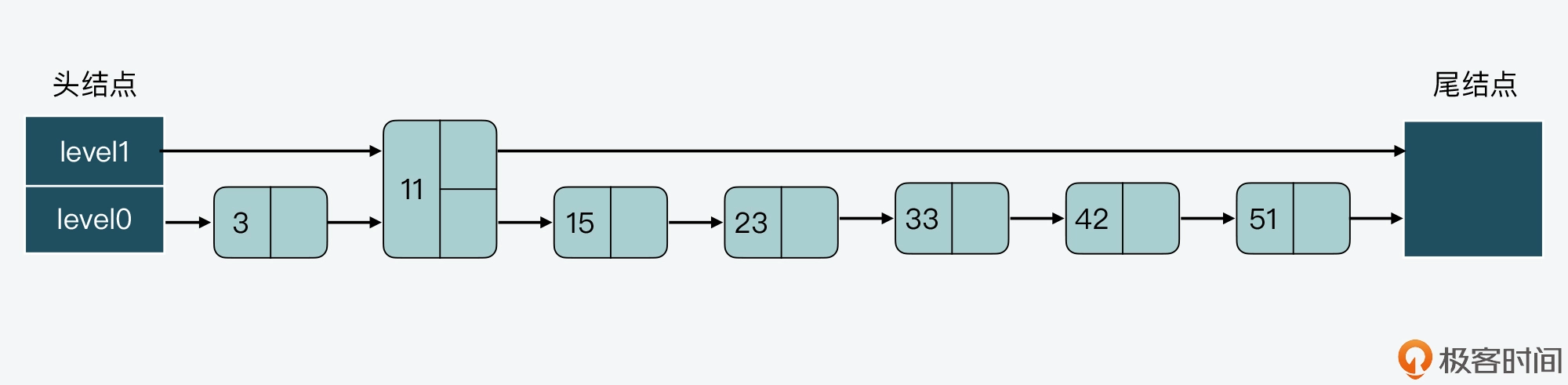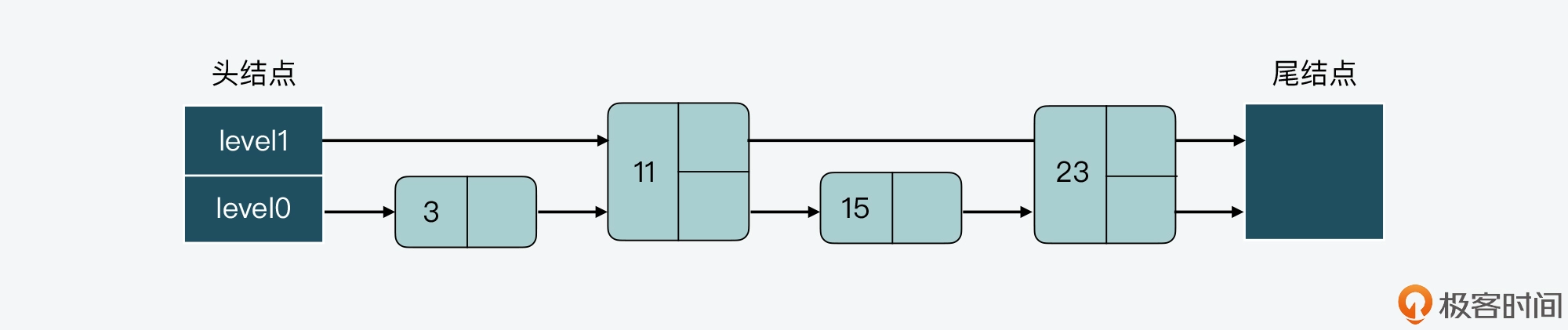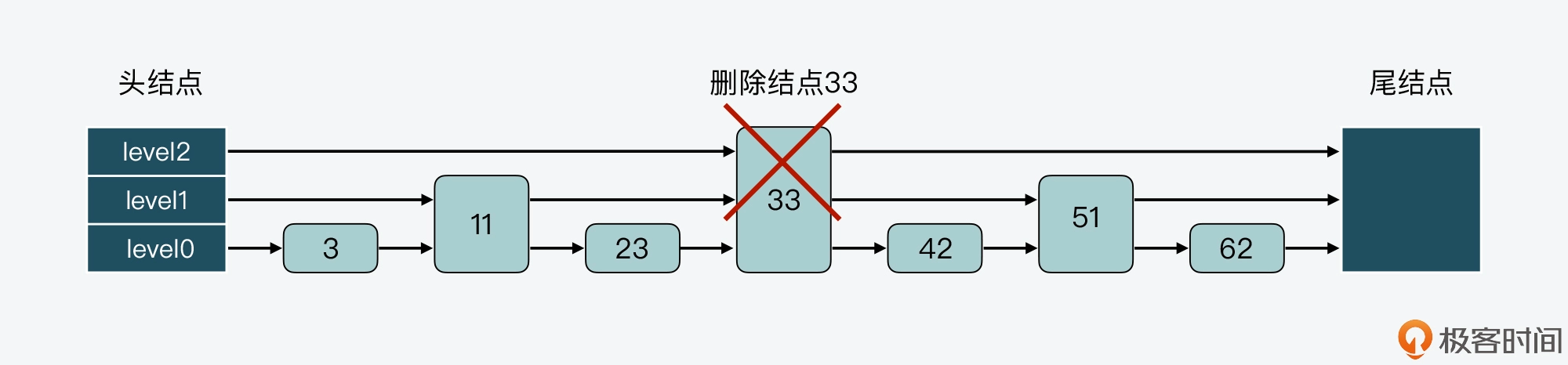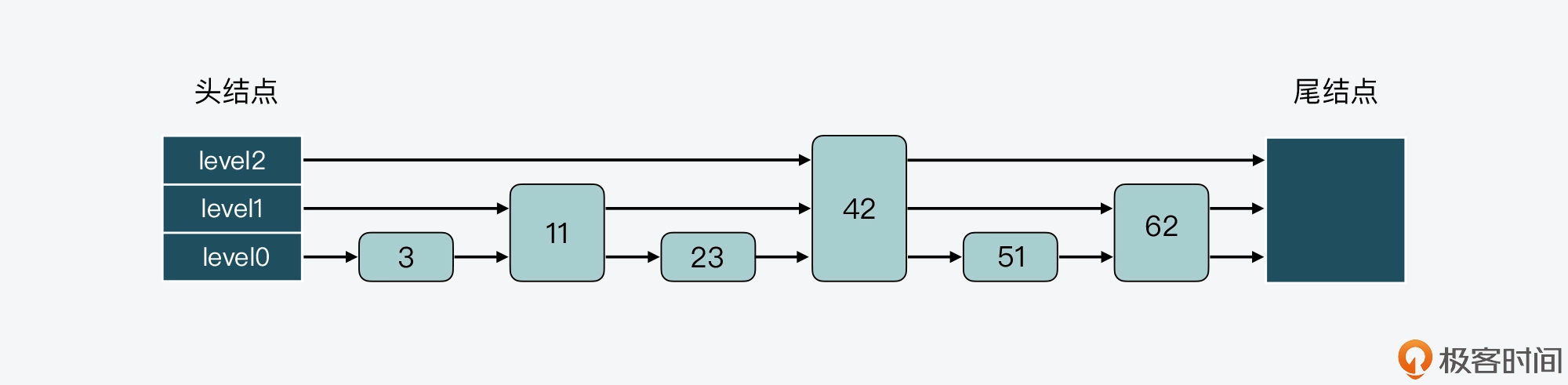``````#define ZSKIPLIST_MAXLEVEL 64  //最大层数为64
#define ZSKIPLIST_P 0.25       //随机数的值为0.25
int zslRandomLevel(void) {
//初始化层为1
int level = 1;
while ((random()&0xFFFF) < (ZSKIPLIST_P * 0xFFFF))
level += 1;
return (level<ZSKIPLIST_MAXLEVEL) ? level : ZSKIPLIST_MAXLEVEL;
}
``````

## 哈希表和跳表的组合使用

`````` zs = zmalloc(sizeof(*zs));
zs->dict = dictCreate(&zsetDictType,NULL);
zs->zsl = zslCreate();
``````

`````` //如果采用ziplist编码方式时，zsetAdd函数的处理逻辑
if (zobj->encoding == OBJ_ENCODING_ZIPLIST) {
...
}
else if (zobj->encoding == OBJ_ENCODING_SKIPLIST) {
zset *zs = zobj->ptr;
zskiplistNode *znode;
dictEntry *de;
//从哈希表中查询新增元素
de = dictFind(zs->dict,ele);
//如果能查询到该元素
if (de != NULL) {
/* NX? Return, same element already exists. */
if (nx) {
return 1;
}
//从哈希表中查询元素的权重
curscore = *(double*)dictGetVal(de);

//如果要更新元素权重值
if (incr) {
//更新权重值
...
}

//如果权重发生变化了
if (score != curscore) {
//更新跳表结点
//让哈希表元素的值指向跳表结点的权重
dictGetVal(de) = &znode->score;
...
}
return 1;
}
//如果新元素不存在
else if (!xx) {
ele = sdsdup(ele);
//新插入跳表结点
znode = zslInsert(zs->zsl,score,ele);
//新插入哈希表元素
...
return 1;
}
..
``````